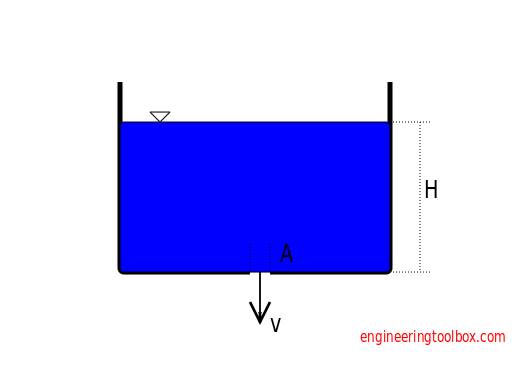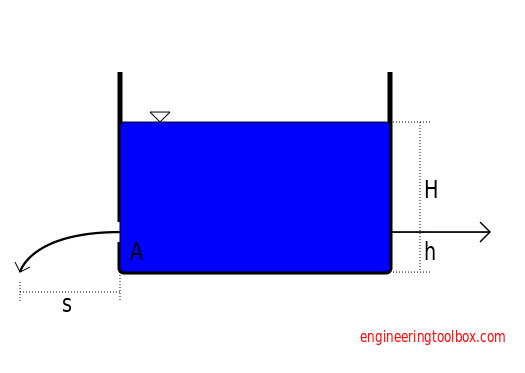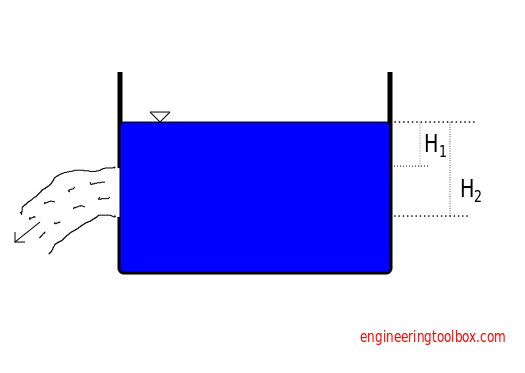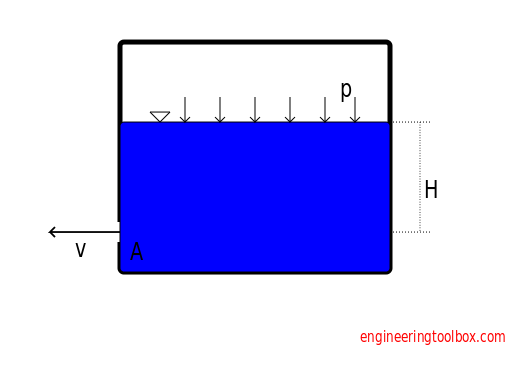Engineering ToolBox - Resources, Tools and Basic Information for Engineering and Design of Technical Applications!

# Liquid Flow from Containers - Emptying Time

## Calculate liquid velocity, volume flow and draining time when emptying a container.

### Base AperturesThe liquid outlet velocity when draining a tank or a container can be calculated

v = Cv (2 g H )1/2                                (1a)

where

v = outlet velocity (m/s)

Cv = velocity coefficient (water 0.97)

g = acceleration of gravity (9.81 m/s2)

H = height (m)

The liquid volume flow can be calculated

V = Cd A (2 g H)1/2                        (1b)

where

V = volume flow (m3/s)

A = area of aperture - flow outlet (m2)

Cd = discharge coefficient

where

Cd = Cc Cv

where

Cc = contraction coefficient (sharp edge aperture 0.62, well rounded aperture 0.97)

A = area aperture (m2)

#### Example - Volume Flow when draining a Container

The height from the surface to the outlet aperture in a water filled container is 3 m. The aperture is sharp edged with diameter 0.1 m. The discharge coefficient can be calculated as

Cd = 0.62 0.97

= 0.6

The area of the aperture can be calculated as

A = π ((0.1 m) / 2)2

=   0.008 m2

The volume flow through the aperture can be calculated as

V = 0.6 (0.008 m2) (2 (9.81 m/s2) (3 m))1/2

= 0.037 m3/s

For height 1.5 m the volume flow is 0.026 m3/s. For height 0.5 m the volume flow is 0.015 m3/s.

### Draining Tank Calculator

This calculator is based on eq. (1b) and can be used to estimate the volume flow and time used to drain a container or tank through an aperture.

The calculator divides the container in "slices" and makes an iterative average calculation for each slice. The accuracy of the calculation can be increased by increasing the number of slices.

- results in table below!

Note! - the flow is reduced and the time is increased with reduced height.

### Small Lateral AperturesOutlet velocity can be expressed as

v = Cv (2 g H)1/2                                (2a)

Distance s can be expressed as

s = 2 (H h)1/2                               (2b)

Volume flow can be expressed as

V = Cd A (2 g H)1/2                             (2c)

Reaction force can be expressed as

F = ρ V v                                 (2d)

where

ρ = density (kg/m3)  (water 1000 kg/m3)

### Large Lateral AperturesVolume flow can be expressed as

V = 2/3 Cd b (2 g)1/2 (H23/2 - H13/2)                                (3a)

where

b = width of aperture (m)

### Excess Pressure in ContainerOutlet velocity can be expressed as

v = Cv (2 (g H + p / ρ))1/2                               (4a)

where

p = excess pressure in container or tank (N/m2, Pa)

Volume flow can be expressed as

V = Cd A (2 (g H + p / ρ))1/2                           (4b)

## Related Topics

• ### Fluid Mechanics

The study of fluids - liquids and gases. Involving velocity, pressure, density and temperature as functions of space and time.

## Related Documents

• ### Bernoulli Equation

Conservation of energy in a non-viscous, incompressible fluid at steady flow.
• ### Cylindrical Tanks - Volumes

Volume in US gallons and liters.
• ### Mechanical Energy Equation vs. Bernoulli Equation

The Mechanical Energy Equation compared to the Extended Bernoulli Equation.
• ### Open Horizontal Pipes - Discharge Length vs. Volume Flow

Discharge length vs. volume flow from water filled horizontal pipes.
• ### Orifice, Nozzle and Venturi Flow Rate Meters

The orifice, nozzle and venturi flow rate meters makes the use of the Bernoulli Equation to calculate fluid flow rate using pressure difference through obstructions in the flow.
• ### Vertical Pipes - Discharge Height vs. Volume Flow

Volume flow and discharge height from vertical water pipes.

## Engineering ToolBox - SketchUp Extension - Online 3D modeling!

Add standard and customized parametric components - like flange beams, lumbers, piping, stairs and more - to your Sketchup model with the Engineering ToolBox - SketchUp Extension - enabled for use with older versions of the amazing SketchUp Make and the newer "up to date" SketchUp Pro . Add the Engineering ToolBox extension to your SketchUp Make/Pro from the Extension Warehouse !

We don't collect information from our users. More about

## Citation

• The Engineering ToolBox (2011). Liquid Flow from Containers - Emptying Time. [online] Available at: https://www.engineeringtoolbox.com/flow-liquid-water-tank-d_1753.html [Accessed Day Month Year].

Modify the access date according your visit.

9.29.12